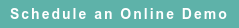# RoyaltyStat Blog

### Posts by Topic

Transfer pricing tax compliance is devoid of external CUP (comparable uncontrolled prices). Therefore, we must select (under the TNMM and under the Profit Split Method) the most appropriate “net” profit indicator (NPI) from comparable uncontrolled enterprises. Most appropriate is the most reliable among competing profit indicators. In economics and statistics, reliability is measured by the coefficient of variation (standard deviation / mean) of the selected variable.

Here is the OECD definition of NPI: “The ratio of net profit to an appropriate base (e.g. costs, sales, assets). The transactional net margin method [TNMM] relies on a comparison of an appropriate net profit indicator for the controlled transaction with the same net profit indicator in comparable uncontrolled transactions.” OECD (2017), § B.3.3 (Determination of net profit). See OECD Transfer Pricing Guidelines for Multinational Enterprises and Tax Administrations 2017, OECD Publishing, Paris, located at: http://dx.doi.org/10.1787/tpg-2017-en

In transfer pricing analysis, one important issue of contention is if, prima facie, asset return (OECD lingo: “return on assets”) is more reliable than profit margin. Lovers of asset return argue that because this NPI includes two multiplicative components, profit margin and asset turnover, asset return provides more financial information than profit margin about comparable enterprises. We show that this purported à priori advantage of asset return over profit margin is not supported by a comparison of their coefficients of variation (measure of reliability).

From the DuPont profit identity, define R = [M ∙ T] = asset return, where M = profit margin (operating profit / revenue) and T = asset turnover (revenue / asset). Define random variable R with mean µR and standard deviation σR, M with mean µM and standard deviation σM, and T with mean µT and standard deviation σT.

Here is a major problem in selecting the most appropriate NPI: If M and T are independent variables, find E[M ∙ T] and Var[M ∙ T]. These two statistical measures are important to determine the coefficient of variation.

We must select the NPI with the smallest coefficient of variation, i.e., we must select the most reliable NPI computed from uncontrolled comparable enterprises:

(1)  If M and T are independent variables, E[R] = E[M ∙ T] = µ= µM µT . However, the variance of R is complex to evaluate because it shows compound errors.

(2)  Var[M ∙ T] = E[M2 T2] – {E[M]E[T]}2 = σR2

= (µM2 + σM2) (µT2 + σT2) − µM2 µT2

= σM2 σT2 + µM2 µT2 + σM2 µT2 + σT2 µM2 − µM2 µT2

σR2 = σM2 σT2 + σM2 µT2 + σT2 µM2

The standard deviation is the square root of (2). See product of independent variables (without our statistical details): https://en.wikipedia.org/wiki/Variance

We can show that the coefficient of variation of M can’t exceed that of R = M ∙ T; therefore, if M and T are independent variables, profit margin is more reliable than asset return.

We define the coefficient of variation of random variable X (which can be M, T or R) as:

(3)  KX = √(σX2 / µX2)

where, like (1) and (2), we have these two statistical parameters defined as:

(4)  µR = µM µT and

(5)  σR2 = σM2 σT2 + σM2 µT2 + σT2 µM2

It follows that the coefficient of variation of asset return is computed with formula:

(6)  KR2 = (σR2 / µR2) = {σM2 σT2 + σM2 µT2 + σT2 µM2} / µM2 µT2

From (6), and the definition of the coefficient of variation in (3), we obtain:

(7)  KR2 = {KM2 (KT2 + 1) +  KT2} > KM2

Therefore, we find our basic result that the coefficient of variation of R is greater than of M:

(8)  KR > KM because KT2 > 0.

In selecting NPI, inequality (8) shows that if M and T are independent variables, profit margin is more reliable than asset return.

Profit margin has several additional advantages over asset return as a more reliable NPI to determine arm's length taxable profits. First, profit margin is a pure number (it has no dimension). Second, asset return suffers from differences in asset vintages or bases (different historical costs of asset acquisition among different comparable enterprises). Third, asset (denominator of asset return) suffers from differences in depreciation rates. See OECD (2017), ¶ 2.104.

Takeaway: If profit margin (M) and asset turnover (T) are independent variables, we have a strong statistical reason to use profit margin instead of asset return as the most reliable (most appropriate) NPI in transfer pricing compliance.

Published on Oct 22, 2018 7:31:38 AM

Ednaldo Silva (Ph.D.) is founder and managing director of RoyaltyStat. He helped draft the US transfer pricing regulations and developed the comparable profits method called TNNM by the OECD. He can be contacted at: esilva@royaltystat.com

RoyaltyStat provides premier online databases of royalty rates extracted from unredacted license agreements
and normalized company financials (income statement, balance sheet, cash flow). We provide high-quality data, built-in analytical tools, customer training and attentive technical support.Topics: Net Profit Indicator